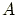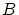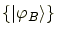# Reduced density operator

RDO(bit labels);
outputs the reduced density operator of the qubits specified by bit labels. To show each vector in the output of the reduced density operator in the format of binary digits, you may command
RDOb(bit labels);

Similarly,

RDOsave (bit labels, file name);
outputs the reduced density operator of the qubits specified by bit labels to the file of file name. Further analysis with the saved matrix should be performed in another calculator such as Octave. This is possible when a user converts it to the matrix in another format (See Sec. 5.3).

To save the reduced density operator of specified qubits into a temporary file, use the command

RDOtmp (bit labels, file name);

Note: These four commands does not change the current state in calculation. They only show or save a reduced density operator.

In general, a reduced density operator of specified qubits () is given by(9)

whereare all the bit strings of the qubits other than.

Let the component systemcomprise the specified qubits, and the component systemcomprise the other qubits. Then the reduced density operator ofis defined as(10)

whereis a complete orthonormal basis of(the Hilbert space of).

Subsections
root
2004-11-07